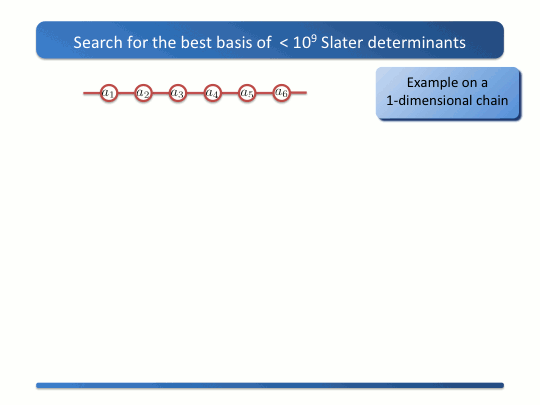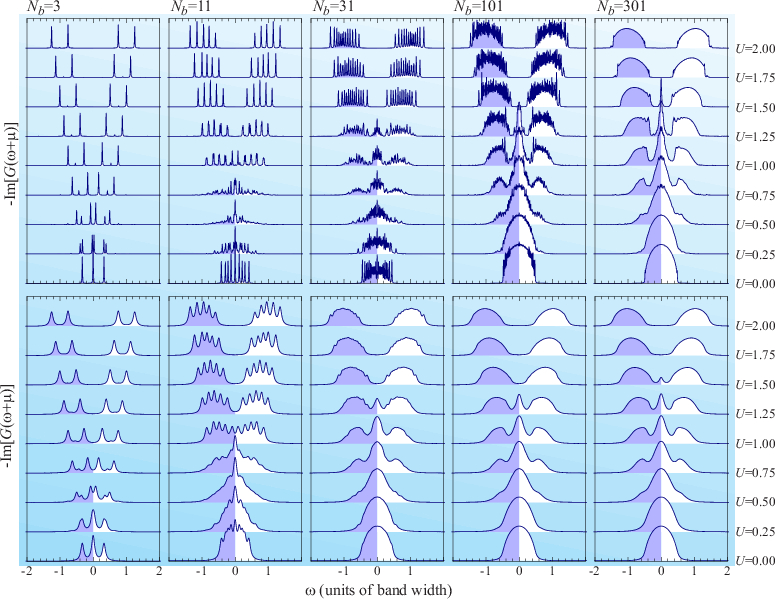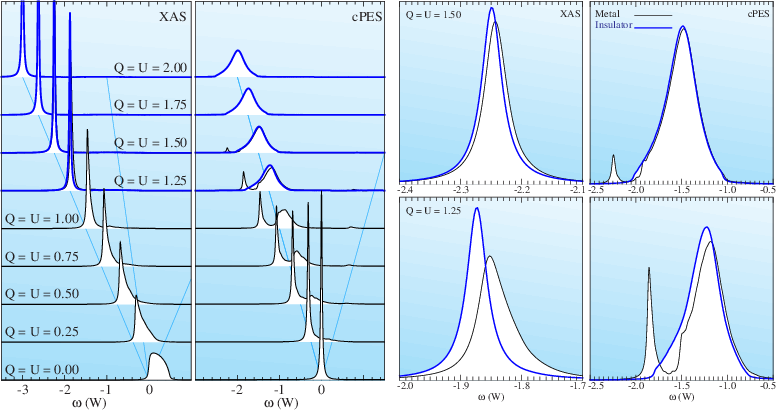# New theoretical methods for correlated electron systems

The theoretical understanding of correlated electron systems is often hindered by the exponential scaling of the computation time and memory required to store the solution as a function of system size. For systems where the local density or Hartree Fock approximations fail, there exists a real computational challenge. Correlated electrons in a quantum many body system are entangled with each other. If one removes one electron from such a system, all the other electrons will feel this and react to it. The wave-function describing such a state cannot be expressed as a time independent product of one-particle orbitals, (single Slater determinant) but must be written as a coherent sum of many of such product states (multiple Slater determinants). The number of Slater determinants needed scales non-polynomial with system size. Obtaining quantum chemical ab initio solutions is impossible for many systems. Even small systems containing only three or four open d- or f-shell ions can be too large to compute. How badly the number of possible determinants scales with system size is illustrated in Fig. 1.FIG. 1: The number of Determinants (right column) grows non-polynomial as a function of system size for Quantum many body calculations. We exemplify this by calculating the total number of determinants possible for a Hubbard model (a lattice of H atoms with only one s-orbital per atom) at half filling as a function of the number of lattice sites.

We have developed a new method for the ab-initio description of the ground-state, excitations, and dynamics of materials with a variable amount of correlation [Haverkort2012,Lu2014]. The method is implemented in the program package Quanty and available free of charge. The method is based on ideas obtained both from quantum chemistry and density matrix renormalization group theory. One should realize that not all determinants possible are equally important. For 1023 particles in a box one can safely assume that they will never be all in one corner at the same time. Our method therefore neglects such improbable states making the quantum many body problem tractable, while keeping the most important effects of entanglement between the electrons.

The question on how many determinants are important highly depends on how one represents the one-particle orbitals. For a bulk solid without many body interactions the many-particle eigenstates are given by single products of Bloch-waves. The ground-state is the state where all Bloch-states with energies lower than the Fermi-energy are occupied. If one would represent the many body states using a local orbital basis one needs an exponential growing number of determinants with system size. For particles only interacting with an effective potential (local density or Hartree-Fock approximation) the optimal one-particle basis is given by the eigenstates of a single electron in that potential. For correlated electron systems the most optimal representation is not a priory known. We therefore at each step in the calculation determine an optimized representation of the one particle orbitals by requiring that the density matrix is diagonal. If Fig. 2 we exemplify this using a one-dimensional chain.FIG. 2: Steps taken in the iterative method. We find the maximal 109 determinants that describe the state at each time step best optimizing both the many-body wave-functions as well as the one particle orbitals. For more information see [Haverkort2012] and [Lu2014].

This method is highly effective for materials which contain elements with different amount of correlations. In transition metal or rare-earth compounds the d- or f-shell is much more localized and correlated than the Ligand p-shells. The same is true in dynamical mean-field theory where one needs to solve an Anderson impurity model in which only the impurity orbitals are correlated and the bath is treated on a mean-field level. Furthermore because we can update the basis during the calculation we are able to calculate dynamics, excitations and spectroscopy.

As a first test we applied our method to dynamical mean-field theory and showed that we are able to reproduce the spectral function of a single band Hubbard model on a Bethe Lattice as previously obtained with Quantum Monte Carlo or numerical renormalization group theory [Lu2014]. The advantage of our method is that we, as other exact diagonalization implementations of dynamical mean-field theory, can work entirely on the real axes preventing the need to do an ill posed analytical continuation of the one particle Green’s function involved. The difference to other exact diagonalization implementations is that we can handle several hundreds of bath sites. We can handle a much finer discretization mesh of the bath, allowing for continues representations of the bands involved. Fig. 3 shows the results as a function of number of bath sites included in the Anderson impurity model. The spectra become less discrete when more bath sites are included, but also the critical where the metal to insulator transition happens changes as a number of discretization energies of the bath.FIG. 3: All panels show the DMFT impurity Greens function for different values of U ranging from 0 to 2 in steps of 0.25 in units of the bandwidth of G0. The different columns show the spectral function for 3, 11, 31, 101, or 301 poles in the bath Greens function and thus sites in the Anderson impurity calculation and therefore respectively 36, 8.5 105, 3.6 1017, 1.6 10 59179 possible determinants in the many body problem. The impurity Greens function in all calculations consists of 1000 poles. The panels in the top row show the spectral functions broadened with a Lorentzian of full width at half maximum 0.01. The panels in the bottom row show the same spectral functions as the top row, but broadened with a Lorentzian of full width at half maximum 0.1. For more information see [Lu2014].

In a second example we looked at excitations as one would measure with core-level x-ray absorption or core-level photoemission across the metal to insulator transition for a single band Hubbard model [Haverkort2014]. This allows us to describe band-transitions, resonances and excitons within a single framework for all different interaction strengths. Fig. 4 shows these spectra for different interaction strengths going through the metal insulator transition.FIG. 4: X-ray absorption and core level photoemission for a single band Hubbard model solved within the dynamical mean field approximation as a function of the valence-valence Coulomb repulsion U and the core-valence Coulomb repulsion Q. The spectra of metallic ground-states are plotted with thin black lines, of insulating ground-states with thick blue lines. For metallic ground-states one can see the dynamical screening of the core hole and its influence on the spectral line-shape. For more information see [Haverkort2014].
Go to Editor View